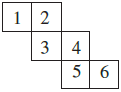Q

# Can this be a net for a die ? Explain your answer. Exercise 15.1 Q.no. 3

Q. 3.     Can this be a net for a die? Explain your answer.Views

NO,
This can not be a net for a die because the opposite faces of a die always have a total sum of 7.
Now, this case one pair is  4  opposite to 1
So, their sum is equal to  5  and not equal to 7
And also, the 2nd pair is 6  opposite to 3 .
So, their sum is equal to 9 and not equal to 7

Exams
Articles
Questions